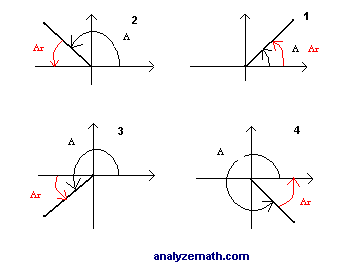# Find Reference Angle

What is the reference angle to an angle in standard position? If A is an angle in standard position, its reference angle Ar is the acute angle formed by the x axis and the terminal side of angle A. See figure below.Two or more coterminal angles have the same reference angle.
Assume angle A is positive and less than 360� (2π), we have 4 possible cases:
1. If angle A is in quadrant I then the reference angle
A r = A .
2. If angle A is in quadrant II then the reference angle
A r = 180� - A if A is given degrees and A r = π - A if A is given in radians.
3. If angle A is in quadrant III then the reference angle
A r = A - 180� if A is given degrees and A r = A - π if A is given in radians.
4. If angle A is in quadrant IV then the reference angle
A r = 360� - A if A is given degrees and A r = 2π - A if A is given in radians.

 Example 1: Find the reference angle to angle A = 120 �. Solution to example 1: Angle A is in quadrant II and the reference angle is given by A r = 180� - 120� = 60� Example 2: Find the reference to angle A = - 15 π / 4. Solution to example 2: The given angle is not positive and less than 2π. We can use the positive and less than 2π coterminal Ac to angle A. Ac = - 15 π / 4 + 2 (2 π) = π / 4 Angle A and Ac are coterminal and have the same reference angle. Ac is in quadrant I, therefore A r = A c = π / 4 Example 3: Find the reference angle to angle A = - 30� Solution to example 3: Angle A is negative, in quadrant IV and its absolute value is less than 90�. Hence A r = | -30� | = 30� Exercises:Find the reference angle to angles 1. A = 1620� 2. A = - 29 π / 6 3. A = - π / 7 Solutions to Above Exercises: 1. A r = 25� 2. A r = π / 6 3. A r = π / 7 More references on angles.